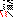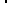# MINLPLib

### A Library of Mixed-Integer and Continuous Nonlinear Programming Instances

#### Instance pooling_bental4pq

`PQ formulation of pooling problem. Explicitly added RLT constraints were removed from the original formulation of Alfaki and Haugland.`
 Formatsⓘ ams gms lp mod nl osil pip py Primal Bounds (infeas ≤ 1e-08)ⓘ -450.00000000 p1 ( gdx sol ) (infeas: 2e-11) Other points (infeas > 1e-08)ⓘ Dual Boundsⓘ -450.00000050 (ANTIGONE)-450.00000050 (BARON)-450.00000000 (COUENNE)-450.00000000 (GUROBI)-450.00000000 (LINDO)-450.00000000 (SCIP) Referencesⓘ Ben-Tal, Aharon, Eiger, Gideon, and Gershovitz, Vladimir, Global minimization by reducing the duality gap, Mathematical Programming, 63:1, 1994, 193-212.Alfaki, Mohammed and Haugland, Dag, Strong formulations for the pooling problem, Journal of Global Optimization, 56:3, 2013, 897-916. Sourceⓘ Bental4.gms from Standard Pooling Problem Instances Applicationⓘ Pooling problem Added to libraryⓘ 12 Sep 2017 Problem typeⓘ QCP #Variablesⓘ 13 #Binary Variablesⓘ 0 #Integer Variablesⓘ 0 #Nonlinear Variablesⓘ 5 #Nonlinear Binary Variablesⓘ 0 #Nonlinear Integer Variablesⓘ 0 Objective Senseⓘ min Objective typeⓘ linear Objective curvatureⓘ linear #Nonzeros in Objectiveⓘ 7 #Nonlinear Nonzeros in Objectiveⓘ 0 #Constraintsⓘ 16 #Linear Constraintsⓘ 10 #Quadratic Constraintsⓘ 6 #Polynomial Constraintsⓘ 0 #Signomial Constraintsⓘ 0 #General Nonlinear Constraintsⓘ 0 Operands in Gen. Nonlin. Functionsⓘ Constraints curvatureⓘ indefinite #Nonzeros in Jacobianⓘ 51 #Nonlinear Nonzeros in Jacobianⓘ 12 #Nonzeros in (Upper-Left) Hessian of Lagrangianⓘ 12 #Nonzeros in Diagonal of Hessian of Lagrangianⓘ 0 #Blocks in Hessian of Lagrangianⓘ 1 Minimal blocksize in Hessian of Lagrangianⓘ 5 Maximal blocksize in Hessian of Lagrangianⓘ 5 Average blocksize in Hessian of Lagrangianⓘ 5.0 #Semicontinuitiesⓘ 0 #Nonlinear Semicontinuitiesⓘ 0 #SOS type 1ⓘ 0 #SOS type 2ⓘ 0 Minimal coefficientⓘ 5.0000e-01 Maximal coefficientⓘ 9.0000e+00 Infeasibility of initial pointⓘ 1 Sparsity JacobianⓘSparsity Hessian of Lagrangianⓘ```\$offlisting
*
*  Equation counts
*      Total        E        G        L        N        X        C        B
*         17        8        0        9        0        0        0        0
*
*  Variable counts
*                   x        b        i      s1s      s2s       sc       si
*      Total     cont   binary  integer     sos1     sos2    scont     sint
*         14       14        0        0        0        0        0        0
*  FX      0
*
*  Nonzero counts
*      Total    const       NL      DLL
*         59       47       12        0
*
*  Solve m using NLP minimizing objvar;

Variables  objvar,x2,x3,x4,x5,x6,x7,x8,x9,x10,x11,x12,x13,x14;

Positive Variables  x2,x3,x4,x5,x6,x7,x8,x9,x10,x11,x12,x13,x14;

Equations  e1,e2,e3,e4,e5,e6,e7,e8,e9,e10,e11,e12,e13,e14,e15,e16,e17;

e1..    objvar - x5 + 5*x6 + 3*x9 + 9*x10 - 6*x11 - 7*x13 - x14 =E= 0;

e2..    x9 + x10 =L= 300;

e3..    x11 + x12 =L= 50;

e4..    x13 + x14 =L= 300;

e5..    x5 + x6 =L= 300;

e6..    x9 + x10 + x11 + x12 + x13 + x14 =L= 300;

e7..    x5 + x9 + x11 + x13 =L= 100;

e8..    x6 + x10 + x12 + x14 =L= 200;

e9..  - 0.5*x5 + 0.5*x9 - 1.5*x11 - 1.5*x13 =L= 0;

e10..    0.5*x6 + 1.5*x10 - 0.5*x12 - 0.5*x14 =L= 0;

e11..    x2 + x3 + x4 =E= 1;

e12.. -x2*x7 + x9 =E= 0;

e13.. -x2*x8 + x10 =E= 0;

e14.. -x3*x7 + x11 =E= 0;

e15.. -x3*x8 + x12 =E= 0;

e16.. -x4*x7 + x13 =E= 0;

e17.. -x4*x8 + x14 =E= 0;

* set non-default bounds
x2.up = 1;
x3.up = 1;
x4.up = 1;
x5.up = 100;
x6.up = 200;
x7.up = 100;
x8.up = 200;
x9.up = 100;
x10.up = 200;
x11.up = 50;
x12.up = 50;
x13.up = 100;
x14.up = 200;

Model m / all /;

m.limrow=0; m.limcol=0;
m.tolproj=0.0;

\$if NOT '%gams.u1%' == '' \$include '%gams.u1%'

\$if not set NLP \$set NLP NLP
Solve m using %NLP% minimizing objvar;

```

Last updated: 2023-06-01 Git hash: fdbb0df7